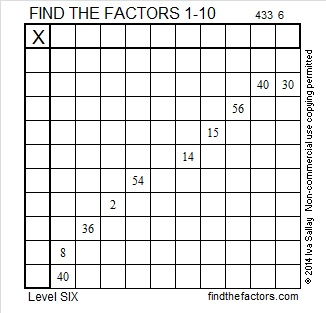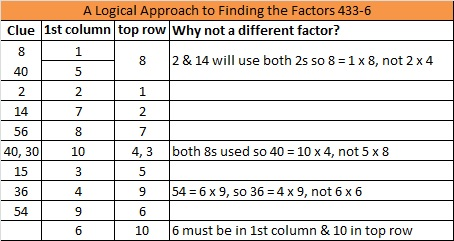# 212 and Level 6

• 212 is a composite number.
• Prime factorization: 212 = 2 x 2 x 53, which can be written (2^2) x 53
• The exponents in the prime factorization are 2 and 1. Adding one to each and multiplying we get (2 + 1)(1 + 1) = 3 x 2 = 6. Therefore 212 has 6 factors.
• Factors of 212: 1, 2, 4, 53, 106, 212
• Factor pairs: 212 = 1 x 212, 2 x 106, or 4 x 53
• Taking the factor pair with the largest square number factor, we get √212 = (√4)(√53) = 2√58 ≈ 14.5602Excel file of puzzles and previous week’s factor solutions: 10 Factors 2014-08-18This site uses Akismet to reduce spam. Learn how your comment data is processed.